2019-04-16 09:19:56 weixin_41791349 阅读数 3193
• ###### C语言位操作视频精讲-C语言专题第二部分

本课程的目标是让大家彻底掌握C语言中位运算符及常规技巧，这些知识在嵌入式代码中用的很多，尤其是设置寄存器以操控硬件时，很多同学被卡在这里。

13627 人正在学习 去看看 朱有鹏

<本文翻译自matlab帮助文档，算是自己对该方法的一点理解和总结>

(在Matlab2018a中用LSTM网络模型进行时间序列预测的方法有两种。

比如已知[x1,x2,...,x10]这个序列，用[x1,x2,...,x9]作为输入，得到[x2,x3,...,x10]，



比如已知[x1,x2,...,x10,x11,...,x20]这个序列，用[x1,x2,...,x9]作为输入，得到[x2,x3,...,x10]，



)

1. 下载序列数据
该序列中的时间步长代表月份，值对应样本数量。把数据集变成一个行向量。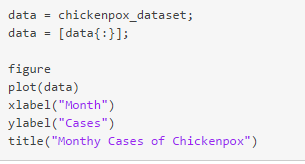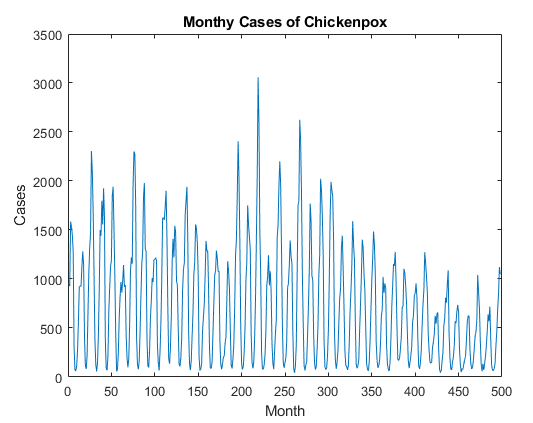把数据集分解：训练占90%，测试占10%。同时，按上文介绍，网络的响应是训练序列移动一个时间步长的值。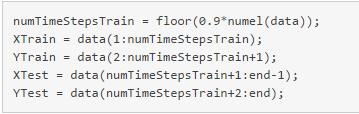2. 标准化数据
为了更好地拟合并防止训练时发散，将训练数据标准化为零均值和单位方差。使用同样的参数格式化测试数据。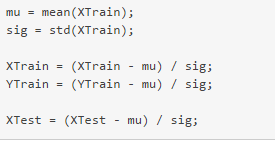3. 定义LSTM网络
创建一个LSTM回归网络，指定LSTM层有200个隐藏神经元。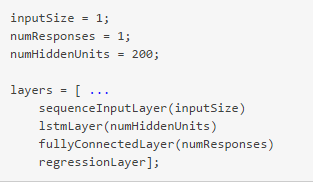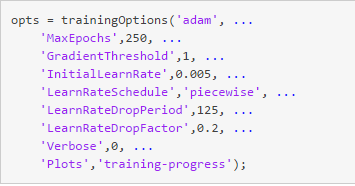4. 训练LSTM网络
使用trainNetwork和指定的训练参数来训练LSTM网络。5. 预测未来值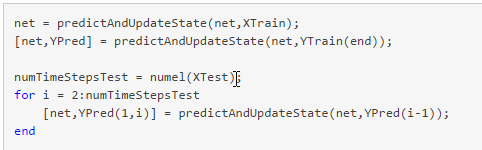2019-07-11 19:40:37 Fraps_ 阅读数 19
• ###### C语言位操作视频精讲-C语言专题第二部分

本课程的目标是让大家彻底掌握C语言中位运算符及常规技巧，这些知识在嵌入式代码中用的很多，尤其是设置寄存器以操控硬件时，很多同学被卡在这里。

13627 人正在学习 去看看 朱有鹏

## 线性相关与生成子空间

### 向量与向量组

$a=\left[ \begin{matrix} \mathrm{a_1} \\ \mathrm{a_2}\\ \vdots \\ \mathrm{a_n} \\ \end{matrix} \right]$

$A=(a_1,a_2,\cdots,a_n)$

### 线性组合

$k_1a_1+k_2a_2+\cdots+k_na_n$称为向量组A的一个线性组合，这组实数称为这个线性组合的系数。

## 实例：主成分分析

2019-10-19 19:59:39 unchainedmelody 阅读数 6
• ###### C语言位操作视频精讲-C语言专题第二部分

本课程的目标是让大家彻底掌握C语言中位运算符及常规技巧，这些知识在嵌入式代码中用的很多，尤其是设置寄存器以操控硬件时，很多同学被卡在这里。

13627 人正在学习 去看看 朱有鹏

# 1、从视频中截取图片并打标签

import os
import numpy as np
import cv2

def mkdir(path):
# 去除首位空格
path = path.strip()
# 去除尾部 \ 符号
# path = path.rstrip("\\")
# 判断路径是否存在
# 存在     True
# 不存在   False
is_exists = os.path.exists(path)
# 判断结果
if not is_exists:
# 如果不存在则创建目录
# 创建目录操作函数
os.makedirs(path)
print(path + ' 创建成功')
return True
else:
# 如果目录存在则不创建，并提示目录已存在
print(path + ' 目录已存在')
return False

def get_all_video_file_name(file_path: str):
"""
:parameter:
file_path: 视频文件路径
:return:
video_files：返回所有视频文件名
"""
video_files_path = []
all_files = []
iter_files(file_path, all_files)
for f in all_files:
if is_video_file(f):
video_files_path.append(f)
return video_files_path

def iter_files(root_dir, all_files=[]):
for root, dirs, files in os.walk(root_dir):
for file in files:
file_name = os.path.join(root, file)
all_files.append(file_name)

def is_video_file(file):
suffix = os.path.splitext(file)
if suffix == '.mp4' or suffix == '.mkv' or suffix == '.wmv' \
or suffix == '.avi' or suffix == '.mpg':
return True
return False

def rotate_bound(image, angle):
# grab the dimensions of the image and then determine the
# center
(h, w) = image.shape[:2]
(cX, cY) = (w // 2, h // 2)
# grab the rotation matrix (applying the negative of the
# angle to rotate clockwise), then grab the sine and cosine
# (i.e., the rotation components of the matrix)
M = cv2.getRotationMatrix2D((cX, cY), -angle, 1.0)
cos = np.abs(M[0, 0])
sin = np.abs(M[0, 1])
# compute the new bounding dimensions of the image
nW = int((h * sin) + (w * cos))
nH = int((h * cos) + (w * sin))
# adjust the rotation matrix to take into account translation
M[0, 2] += (nW / 2) - cX
M[1, 2] += (nH / 2) - cY

# perform the actual rotation and return the image
return cv2.warpAffine(image, M, (nW, nH))

def interception_frame_by_file_name(input_video_path: str, output_img_path: str, times_frame: int, video_area=None):
"""
视频文件以label命名
:parameter:
input_video_path: 要处理的视频文件路径
output_video_path: 输出路径
video_area：截取的区域，list类型，如 [row_start,row_end,col_start,col_end]
timesFrame: 间隔多少帧取一帧
:return:
"""

if video_area is None:
video_area = [150, 150 + 64, 300, 300 + 64]

file_name_arr = get_all_video_file_name(input_video_path)
cnt = 1  # 当前截取的图片个数
# 使用opencv按一定间隔截取视频帧，并保存为图片
for file_path in file_name_arr:
print('正在处理' + file_path)

# 创建相关文件夹
folder_name = str.split(file_path, '\\')[-1][:-4]
dif_img_path = output_img_path + '\\differenceImage\\' + folder_name
static_img_path = output_img_path + '\\staticImage\\' + folder_name
mkdir(dif_img_path)
mkdir(static_img_path)

vc = cv2.VideoCapture(file_path)  # 读取视频文件
c = 1  # 计数器，记录当前的帧数

if vc.isOpened():  # 判断是否正常打开
else:
rval = False
frame_prev = None
while rval:  # 循环读取视频帧
if c % times_frame == 0:  # 每隔timeF帧进行存储操作
frame = frame[video_area:video_area, video_area:video_area]
cv2.imwrite(static_img_path + '\\' + str(cnt) + '.jpg', frame)  # 存储静态图

if frame_prev:
frame_dif = cv2.absdiff(frame_prev, frame)
cv2.imwrite(dif_img_path + '\\' + str(cnt) + '.jpg', frame_dif)  # 存储残差图

frame_prev = frame
cnt += 1

c = c + 1
cv2.waitKey(1)

vc.release()

def interception_frame(input_video_path: str, output_img_path: str, label_arr: list, times_frame: int, video_area=None):
"""
从视频指定区域截取图片
:parameter:
input_video_path: 要处理的视频文件路径
output_video_path: 输出路径
label_arr: 存放视频文件的label
video_area：截取的区域，list类型，如 [row_start,row_end,col_start,col_end]
timesFrame: 间隔多少帧取一帧
:return:
"""

if video_area is None:
video_area = [150, 150 + 64, 300, 300 + 64]

# 使用opencv按一定间隔截取视频帧，并保存为图片
for j, folder_name in enumerate(label_arr):
cnt = 1  # 当前截取的图片个数

print('正在处理' + str(j + 1) + '.mp4')

dif_img_path = output_img_path + '\\differenceImage\\' + str(folder_name)
static_img_path = output_img_path + '\\staticImage\\' + str(folder_name)
mkdir(dif_img_path)
mkdir(static_img_path)

vc = cv2.VideoCapture(input_video_path + '\\' + str(j + 1) + '.mp4')  # 读取视频文件
c = 1  # 计数器，记录当前的帧数

if vc.isOpened():  # 判断是否正常打开
else:
rval = False
frame_prev = np.array([])
while rval:  # 循环读取视频帧

if c % times_frame == 0 and rval:  # 每隔timeF帧进行存储操作
frame = frame[video_area:video_area, video_area:video_area]
cv2.imwrite(static_img_path + '\\' + str(cnt) + '.jpg', frame)  # 存储静态图

if len(frame_prev) > 0:
frame_dif = cv2.absdiff(frame_prev, frame)
cv2.imwrite(dif_img_path + '\\' + str(cnt) + '.jpg', frame_dif)  # 存储残差图

frame_prev = frame
cnt += 1

c = c + 1
cv2.waitKey(1)

vc.release()

# 处理训练集
input_video_path = r'E:\research\beihaivideo\train'
output_img_path = r'E:\research\beihaivideo\train'
label_arr = [7, 6, 6, 5, 5, 2, 2, 3, 3]
times_frame = 12
video_area = None
interception_frame(input_video_path, output_img_path, label_arr, times_frame)

# # 处理测试集
# input_video_path = r'E:\research\beihaivideo\test'
# output_img_path = r'E:\research\beihaivideo\test'
# label_arr = [6, 5, 2, 3]
# times_frame = 12
# video_area = None
# interception_frame(input_video_path,output_img_path,label_arr,times_frame)



# 2、从文件夹中分批读取图像，供模型使用

import tensorflow as tf
import os

def read_image(root_path: str, batch_size: int, shape: tuple, shuffle=True):
"""tensorflow 用队列读取大量图片
将root_path下所有图片(.jpg)以batch_size分块读取；
同一label的图片放在同一个文件夹里，文件夹以label命名
:param root_path: 要读取的图片放在此文件夹下
:param batch_size:
:param shape: (height，width，channels)
:param shuffle:
:return:
"""
(img_height, img_width, img_channels) = shape

image_paths_list = []
labels_list = []
classes_list = sorted(os.walk(root_path).__next__())
for c in classes_list:
c_dir = os.path.join(root_path, c)
walk = os.walk(c_dir).__next__()
for sample in walk:
if sample.endswith('.jpg') or sample.endswith('.jpeg'):
image_paths_list.append(os.path.join(c_dir, sample))
labels_list.append(int(c))

# 将img_paths_list 和 labels_list 转换为tf可以处理的格式
image_paths_list = tf.convert_to_tensor(image_paths_list, tf.string)
labels_list = tf.convert_to_tensor(labels_list, tf.int32)
# 建立 Queue, num_epochs=None,生成器可以无限次遍历tensor列表，如果设置为 num_epochs=N，生成器只遍历tensor列表N次。
img_path, label = tf.train.slice_input_producer([image_paths_list, labels_list], shuffle=shuffle, num_epochs=1)

# 读取图片，并进行解码，处理其他格式的图片则需要修改此处
image = tf.image.decode_jpeg(image, channels=img_channels)

# 对图片进行裁剪和正则化（将数值[0,255]转化为[-1,1]）
image = tf.image.resize_images(image, size=[img_height, img_width])
image = image * 1.0 / 127.5 - 1.0

# 创建 batch
X, Y = tf.train.batch([image, label], batch_size=batch_size, num_threads=1, capacity=batch_size * 4)
return X, Y



2019-04-15 15:43:07 selinaqqqq 阅读数 432
• ###### C语言位操作视频精讲-C语言专题第二部分

本课程的目标是让大家彻底掌握C语言中位运算符及常规技巧，这些知识在嵌入式代码中用的很多，尤其是设置寄存器以操控硬件时，很多同学被卡在这里。

13627 人正在学习 去看看 朱有鹏

现有的几种深度学习的框架有：caffe，tensorflow，keras，pytorch以及MXNet，Theano等，可能在工业界比较主流的是tensorflow，而由于pytorch比较灵活所以在科研中用的比较多。本文算是对我这两年来使用各大框架的一个总结，仅供参考。

大四的时候接触到的深度学习，那时候的毕设题目是基于深度学习的图像处理，我采用CNN处理进行细粒度的图像检索，把CNN改进成了双向的Bi-CNN。那时候正是caffe大火的时候，在图像处理领域尤其是CNN上确实十分吃香。但是caffe设计的时候就是针对图像，对于一些文本，流数据，时间数据的处理效果不好，所以caffe也只用过这一次。

使用caffe的流程
#第一步

#第二步

#第三步

#第四步

#第五步

Tensorflow的体系就比较灵活，能够使用单个API将计算部署到服务器或移动设备中的某个或多个CPU或GPU上。然后研一上自然语言处理的时候需要做实体命名识别的作业，提到中文命名实体识别就会想到BiLSTM+CRF，github上的代码是基于tensorflow的，这是第一次使用tensorflow搭建模型。

使用Tensorflow搭建神经网络主要包含以下6个步骤
#定义添加神经层的函数
#准备训练的数据
#定义节点准备接收数据
#定义神经层：隐藏层和预测层
#定义loss表达式
#选择optimizer使loss达到最小
#对所有变量进行初始化，通过sess.run optimizer，迭代多次进行学习



Keras由纯Python编写而成并基于Tensorflow、Theano以及CNTK后端，相当于Tensorflow、Theano、CNTK的上层接口，号称10行代码搭建神经网络，具有操作简单、上手容易、文档资料丰富、环境配置容易等优点，简化了神经网络构建代码编写的难度。目前封装有全连接网络、卷积神经网络、RNN和LSTM等算法。

#第一步：选择模型
model = Sequential()

#第二步：构建网络层

#第三步：编译
sgd = SGD(lr=0.01, decay=1e-6, momentum=0.9, nesterov=True) # 优化函数，设定学习率（lr）等参数
model.compile(loss='categorical_crossentropy', optimizer=sgd, class_mode='categorical') # 使用交叉熵作为loss函数

'''
#第四步：训练
.fit的一些参数
batch_size：对总的样本数进行分组，每组包含的样本数量
epochs ：训练次数
shuffle：是否把数据随机打乱之后再进行训练
validation_split：拿出百分之多少用来做交叉验证
verbose：屏显模式 0：不输出  1：输出进度  2：输出每次的训练结果
'''
(X_train, y_train), (X_test, y_test) = mnist.load_data() # 使用Keras自带的mnist工具读取数据（第一次需要联网）

#由于mist的输入数据维度是(num, 28, 28)，这里需要把后面的维度直接拼起来变成784维
X_train = X_train.reshape(X_train.shape, X_train.shape * X_train.shape)
X_test = X_test.reshape(X_test.shape, X_test.shape * X_test.shape)
Y_train = (numpy.arange(10) == y_train[:, None]).astype(int)
Y_test = (numpy.arange(10) == y_test[:, None]).astype(int)
model.fit(X_train,Y_train,batch_size=200,epochs=50,shuffle=True,verbose=0,validation_split=0.3)
model.evaluate(X_test, Y_test, batch_size=200, verbose=0)

#第五步：输出
print("test set")
scores = model.evaluate(X_test,Y_test,batch_size=200,verbose=0)
print("")
print("The test loss is %f" % scores)
result = model.predict(X_test,batch_size=200,verbose=0)
result_max = numpy.argmax(result, axis = 1)
test_max = numpy.argmax(Y_test, axis = 1)
result_bool = numpy.equal(result_max, test_max)
true_num = numpy.sum(result_bool)
print("")
print("The accuracy of the model is %f" % (true_num/len(result_bool)))


然后后来接触到GAN，seq-GAN的模型，他们用的都是pytorch，就去搭建了pytorch。pytorch和TensorFlow的一点区别，那就是pytorch是一个动态的框架，而TensorFlow是一个静态的框架。我们知道TensorFlow构建的计算图是不能够变的了，然后我们再传入不同的数据进去，进行计算。这就带来一个问题，就是固定了计算的流程，势必带来了不灵活性，如果我们要改变计算的逻辑，或者随着时间变化的计算逻辑，这样的动态计算TensorFlow是实现不了的，或者是很麻烦。pytorch就可以很好的解决这个问题。不过其实工业应用还是tensorflow比较多，或许是TensorFlow在GPU的分布式计算上更为出色，在数据量巨大时效率比pytorch要高一些，但好像学术科研的时候pytorch用的比较多，可能是pytorch比较灵活。

2018-09-30 22:18:49 crazyeden 阅读数 361
• ###### C语言位操作视频精讲-C语言专题第二部分

本课程的目标是让大家彻底掌握C语言中位运算符及常规技巧，这些知识在嵌入式代码中用的很多，尤其是设置寄存器以操控硬件时，很多同学被卡在这里。

13627 人正在学习 去看看 朱有鹏

a. 在图像中用slective search 方法 确定约1000-2000个候选框

b. 对于每个候选框内图像块，使用深度网络提取特征，得到一个固定长度的特征向量

We extract a 4096-dimensional feature vector from each region proposal using the Caffe implementation of the CNN described by Krizhevsky etal.这里很清楚的说了，作者就是用了AlexNet的网络结构最好提取4096维的一维向量，作为特征向量。

c. 对候选框中提取出的特征，使用SVM二分类器判别是否属于一个特定类

d. 对于属于某一特征的候选框，用回归器进一步调整其位置

1 SVM具体训练实现细节

2 回归框的训练方法